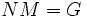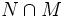# Direct factor versus normal

This survey article compares, and contrasts, the following subgroup properties: direct factor versus normal subgroup
View other subgroup property comparison survey articles$|$ View all comparison survey articles$|$ View survey articles related to direct factor$|$ View survey articles related to normal subgroup

## Introduction

This article is about the relation, the similarity and contrast, between the well-known subgroup property of normality, and the important subgroup property of being a direct factor -- a factor in a direct product.

## Definitions

### Normal subgroup

Further information: normal subgroup

A subgroup$N$ in a group$G$ is termed normal (in symbols,$N \triangleleft G$) if it satisfies the following equivalent conditions:

•$N$ is the kernel of a homomorphism from$G$. In particular, there is a quotient group$G/N$.
• Every inner automorphism of$G$ leaves$N$ invariant. In symbols,$gNg^{-1} = N$ for every$g \in G$.

### Direct factor

Further information: direct factor

A subgroup$N$ of a group$G$ is termed a direct factor if it is normal, and there is another subgroup$M$ of$G$ such that$M$ is normal,$NM = G$ and$N \cap M$ is trivial.

(The three conditions are equivalent to saying that$G$ is the internal direct product of$N$ with$M$. Thus,$G$ is isomorphic to the external direct product$N \times M$).

Such a$M$ will be termed a normal complement to$N$.

## Implication relations

### Direct factor implies normal

Further information: direct factor implies normal

### Normal not implies direct factor

Further information: normal not implies direct factor

## Corresponding notions of simplicity

### Simple group

Further information: simple group

### Directly indecomposable group

Further information: directly indecomposable group

## Similar role in the factorization sense

### Normal subgroups as a tool for factorization

Just as we factorize natural numbers as products of smaller natural numbers, we want to factorize groups in terms of smaller subgroups. One such approach is using normal subgroups. If$N \triangleleft G$, then we can intuitively think of$G$ as being factored into two pieces$N$ and$G/N$.

This analogy, though, fails in the following senses:

1. The role played by$N$ and$G/N$ in the factorization is not symmetric, and in this sense, the factorization does not commute. While$N$ is a subgroup,$G/N$ is a quotient group. There are situations where we can identify$G/N$ with a subgroup of$G$ that intersects$N$ trivially (this is the situation of an internal semidirect product) but this is not always true.
2. The structure of$G$ is not determined by the structure of$N$ and that of$G/N$. In other words, knowing$N$ and$G/N$ upto isomorphism doesn't reveal$G$ upto isomorphism. There is additional pasting information that describes how$N$ and$G/N$ interact. This is in sharp contrast to the case of numbers, where we can multiply the factors together to get back the original number.

One can use factorization in the sense of normal subgroups to get the notion of composition series: a subnormal series with the property that all successive quotient groups are simple. A uniqueness theorem of sorts holds for all groups admitting a composition series of finite length: any two composition series have the same collection of isomorphism types of simple groups, with the same multiplicity of each isomorphism type.

### Direct factors as tools of factorization

Factorization of natural numbers may seem to bear closer resemblance to the notion of direct factors in a group. Namely, if$N$ is a direct factor, with a normal complement$M$, we may think of$G$ as factored into$N$ and$M$.$M$ is isomorphic to the quotient group$G/N$, and$N$ is isomorphic to the quotient group$G/M$, so this is a special case of the general factorization in terms of normal subgroups.

This approach doesn't have the two failings mentioned above for normal subgroups:

1. The role played by$N$ and$M$ is symmetric. Thus, the factorization is commutative.
2.$G$ is completely determined by$N$ and$M$. In fact, it is isomorphic to the external direct product$N \times M$.

On the other hand, knowing$N$ does not completely determine$M$ as a subgroup. Although any normal complement to$N$ must be isomorphic to$G/N$, there could be many different normal complements to$N$.

Direct factors can be used as a tool of factorization. Every finite group is expressible as a direct product of directly indecomposable groups, and the Remak-Schmidt theorem states that the isomorphism types and multiplicities of these pieces are independent of the choice of decomposition.